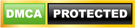Blog

# Python Range

## General Introduction to Python Programming language:

Python is one of the easiest programming languages to either newbies or seasoned professionals alike. If you are completely new to programming language, you should be able to pick up the concepts at a quicker pace and if you already have some coding knowledge (whatever might be the programming language that you have already learnt), learning Python is just a piece of cake.

Want To Get Python Training From Experts? Enroll Now For Free Demo On Python Training

If you’re here then we assume that you are very much interested in getting your hands dirty with the Python programming skills, so why delay? Let us deep dive into the basics of the programming language. Considering that you have enough knowledge on the programming language itself and a little coding skills, let’s check what does the range() function has in store for us all Python developers.

## Python range function:

As a newbie programmer in Python, you should have encountered the range() function amidst your learnings in loop constructs. If you have not encountered it earlier, you are now at the correct place to understand what this function is for and how this can be used at all. The range() function is provided by Python to generate an automatic sequence of integers in the form of a list.
A point to note is that the given end point is never the part of the range that this function generates, as range() function generates a sequence of integers starting from 0 and ends just one integer below the range provided for. Let us take a look at one of the simplest examples to understand what this function does:
The range() function is available in two sets of parameters, as listed below:

Checkout Python Interview Questions

range(stop)
This method will just take the end of the sequence and the function generates the sequence excluding the number specified in the stop input parameter.

range([start],stop[,step])
This method will start the sequence from the start as provided in the function until stop is reached with a user defined step size. The parameter step is an optional parameter, if nothing is provided then by default the step size is picked up as 1.

The example needs a lot of discussion for us to understand the specifics of it.

* All parameters should be integers only
* All parameters can be positive, negative or a combination of both.
* range() function is 0-index based (just as like lists are)
* If the range() function is given a beginning and ending of the sequence that it needs to generate, then it will generate as per input excluding the end number provided in the input to the function.
* If the range() function needs to generate a sequence of numbers not with a step of 1 (which is by default), you can specify this on the function itself and the sequence would be generated as per the step size mentioned.
* If the range() function is not given any beginning number then it by default starts from 0 and this the same that we have tried to display in the example below.

Checkout Python Sample Resumes

Now with these points discussed, the example (below) and the concept should be absolutely clear.Checkout Python Tutorial

Related Articles:
Code Introspection in Python
Python partial functions using functools